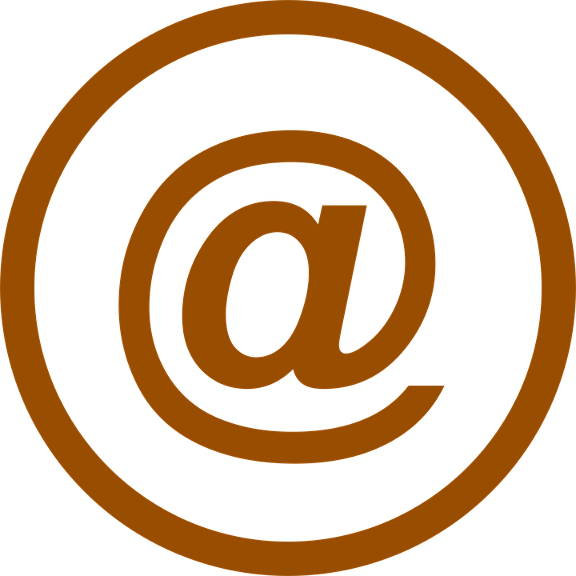HOME > Numerology > Email Address NumerologyIn this century you need to have an email address for your personal use and official uses. We often create an email address without any prior check with the numerology and whatever is our favorite we tend to put them. It also goes for the password. Since it is the combination of names and numbers it is highly critical for it to be perfect and easily accessible and appealing to others. So let us know about the appropriate email address for separate uses.

The basic rules that you should follow while creating any email address are to keep it as simple as possible. You must not include any characters which might create confusion and which are complicated for others. It is the same applied for the password also.
Those who dont know how to calculate or want to do it manually then the following process is for them.

### There are three basic sides of an email address.

• First part is for the name of the person. It is known as the numerology corner stone.
• The second part is the vowels as it is impossible that the email address is without any vowels. It is known as the open heart number.
• The third portion is the combination of your destiny, heart desire, and personality number.

So all the things you have to consider before you create an email account. Now let us take an [email protected]. Now let us do the calculation. Here ABC company initial is thus a is the cornerstone number. Here it is a vowel but it can also be a consonant. Further, the digit has to be converted into numeric character like a = 1.

Now let us take the open heart number by considering the vowels. Here the first vowel is also a. But sometimes there is no vowel in the name then it will not be applicable. Now if the name is having non-alphanumeric characters then they should be removed first of all and then convert them between numbers 1-9. Let us discuss it by drawing a table.

 A = 1 B = 2 C = 3 D = 4 E = 5 F = 6 G = 7 H = 8 I = 9

The next step is to calculate the destiny, heart's desire and the personality number.

 1 2 3 4 5 6 7 8 9 A B C D E F G H I J K L M N O P Q R S T U V W X Y Z

It is the same as you were calculating for your name numerology. The double digits that we should consider are numbers 11 and 22. So we have to find the numbers with the alphabets and add them up so that they are reduced to a single digit or numbers 11 or 22. Then we should stop and consider the result as its destiny number. with the example taken was company ABC here the destiny number will be a=1+b=2+c=3, the total will be 6. So the destiny number is 6.

Now the hearts desire number should be calculated by considering all the vowel like ABC here the only vowel is a=1 thus the hearts desire number is 1.

Then it is the personality number which is the sum of all the consonants in the name ABC. Here b=2 and c=3 so add them up it will give the sum of number 5.

Now let us discuss the effects of each of them one by one.

The corner stone number is the number which is creativity and always welcoming. It always encourages conversation.

Next is the open heart number which is the first vowel of the name and it means social works and the sensitivity towards others.

Now it come to the three numbers the destiny, heart's desire and the personality number. Here in our example, the destiny number is 6 which means harmony, responsibility and at home. Its nature is to take everything at the hype and at a great seriousness. The hearts desire number came as 1 which means leadership and self-confidence. And at last, the personality number is 5 which mean freedom and individuality.

If you want to calculate it automatically then there is also an option for you and see if the email address then you created is going with the calculation or not and if there are any changes required.